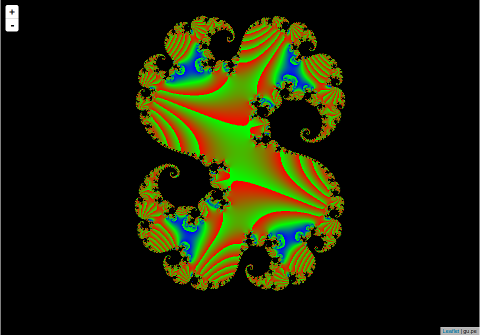## Fractal Slippy Map in golang and LeafletJSRecently I’ve been playing with golang and LeafletJS. As a small project I decided to knock together a fractal slippy map with a golang based map server which dynamically creates the fractal tile images. The whole thing can be reverse proxied via nginx to take the load off the server. The projects totals less than 200 lines of golang as JS and these are my design notes. You can see the final project here and grab the code on github here.

## The HTML

The HTML is extremely simple, the map is a single div:

```<div id="map" style="width: 100px; height: 100px; display: inline-block;"></div>
```

Which is then populated by LeafletJS as follows:

```<script src="leaflet.js"></script>
<script>
document.getElementById('map').style.width  = window.innerWidth-321 + "px";
document.getElementById('map').style.height = window.innerHeight-20 + "px";

var map = L.map('map').setView([-10, -360], 2);

L.tileLayer('http://gu.pe/map/map/{z}/{x}/{y}.png', {
maxZoom: 18,
id: 'example'
</script>
```

The first couple of lines ensure that the map is sized to fill the whole window. The rest just initialises LeafletJS and tells it where to fetch map files from. Map tiles are just simple 256×256 png images.

## The golang server

Both the static html and the dynamically generated tiles are served via golang. Here’s the main function that initializes everything:

```func main() {
http.HandleFunc("/map/", handler)
http.Handle("/", httpgzip.NewHandler(http.FileServer(http.Dir("."))))
http.ListenAndServe(":8080", nil)
}
```

I’m using the httpgzip package which allows golang to server serve gzip compressed content. This wraps the standard golang file server on line 3 above. A hander is registered on /map/ and this is where the png tile images are generated.

```func handler(w http.ResponseWriter, r *http.Request) {

// URLs here look like http://localhost:8080/map/13/4100/2724.png
//                                               z  x    y

tile_size   := 256
tile_size_f := float64(256)

// splits out the URL to get the x,y,z coordinates
spliturl := strings.Split(r.URL.Path, "/")
tile_zi, _ := strconv.Atoi(spliturl)
tile_z := float64(tile_zi)
tile_xi, _ := strconv.Atoi(spliturl)
tile_x := float64(tile_xi)-1
tile_yi, _ := strconv.Atoi(strings.Split(spliturl,"."))
tile_y := float64(tile_yi)-1
fmt.Printf("Input: %f %f %f\n", tile_z,tile_x,tile_y)

myimage := image.NewRGBA(image.Rectangle{image.Point{0,0},image.Point{tile_size,tile_size}})

// This loop just fills the image tile with fractal data
for cx := 0; cx < tile_size; cx++ {
for cy := 0; cy < tile_size; cy++ {

cx_f := float64(cx)
cy_f := float64(cy)

i := complex128(complex(0,1))

zoom := float64(math.Pow(2,float64(tile_z-2)))

tile_range   := 1/zoom
tile_start_x := 1/zoom + (tile_range*tile_x)
tile_start_y := 1/zoom + (tile_range*tile_y)

x := -2 + tile_start_x + (cx_f/tile_size_f)*tile_range
y := -2 + tile_start_y + (cy_f/tile_size_f)*tile_range

// x and y are now in the range ~-2 -> +2

z := complex128(complex(x,0)) + complex128(complex(y,0))*complex128(i)

c := complex(0.274,0.008)
for n := 0; n < 100; n++ {
z = z*z + complex128(c)
}

z = z *10
ratio := float64(2 * (real(z)/2))
r     := math.Max(0, float64(255*(ratio - 1)))
b     := math.Max(0, float64(255*(1 - ratio)))
g     := float64(255 - b - r)
col := color.RGBA{uint8(r),uint8(g),uint8(b),255}
myimage.Set(cx,cy,col)
}
}

png.Encode(w, myimage)
}
```

The bulk of the code is related to the Julia set generation. PNGs are relatively easy to generate and serve in golang, and I previously wrote up my notes on doing that here. The code that splits out the URL is also a mess, I guess something like sscanf would have made a better job of this? However I’m not sure what the canonical way of doing this in golang is.